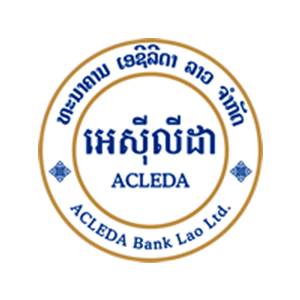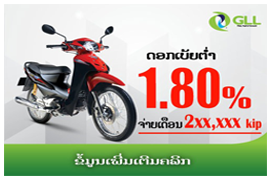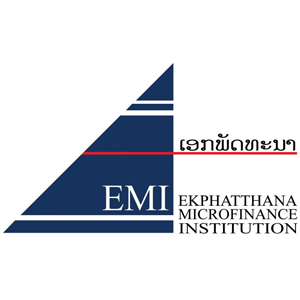Yula.la• Interest 16%
• 6 years• Low interest
• This is testing sample• This is testing sample
• This is testing sample
• This is testing sample• This is testing sample
• This is testing sample
• This is testing sample